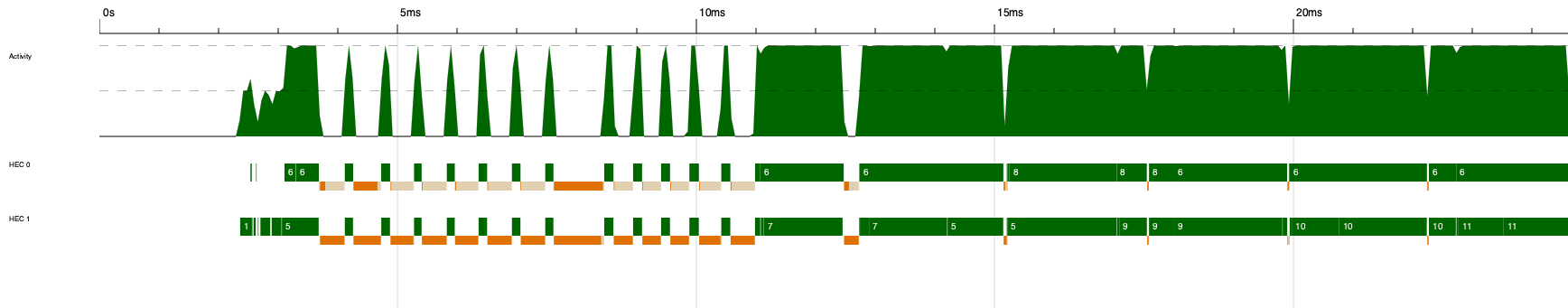In this post I reproduce the haskell parallel teachings and observations found in chapter two of the book Parallel and Concurrent Programming in Haskell by Simon Marlow.

The author uses a sudoku solver to explain how to parallelize cpu bound work using the Eval monad, rpar, rseq while using threadscope to better understand and iterate over the solution.

The source code can be found on github.

The `main` function drives four different implemenations that solve a thousand sudoku puzzles found in the file `sudoku17.1000.txt`.

``````-- Expected cmd line args:
--   filename version
-- The filename containing the puzzles.
-- The version is one of (v1, v2, v3, v4).
main :: IO ()
main = do
f : v : [] <- getArgs
let puzzles   = lines file
solutions = version v puzzles
if v == "v4" then evaluate (length puzzles) else return 0
print (length (filter isJust solutions))

version :: String -> [String] -> [Maybe Sudoku.GridValues]
version "v1" = v1
version "v2" = v2
version "v3" = v3
version "v4" = v3
``````

The implementation of sudoku solver is not important in this experiment. The only relevant piece of information is the `solve` method that receives a String representation of a puzzle and returns a `Maybe Sudoku.GridValues`. The Maybe data structure indicates if a solution has been found. More information about the sudoku solver can be found here.

The first implementation solves the puzzles sequentially.

``````v1 :: [String] -> [Maybe Sudoku.GridValues]
v1 puzzles = map solve puzzles
``````

The second implementation divides the list of puzzles in two and then solves the puzzles of each list in parallel.

``````v2 :: [String] -> [Maybe Sudoku.GridValues]
v2 puzzles =
let (as,bs) = splitAt (length puzzles `div` 2) puzzles in
runEval \$ do
as' <- rpar (force (map solve as))
bs' <- rpar (force (map solve bs))
rseq as'
rseq bs'
return (as' ++ bs')
``````

The third implementation divides the work dynamically leaving to the runtime environment how to better allocate resources and parallelize work.

``````v3 :: [String] -> [Maybe Sudoku.GridValues]
v3 puzzles = runEval (parMap' solve puzzles)

parMap' :: (a -> b) -> [a] -> Eval [b]
parMap' f [] = return []
parMap' f (a:as) = do
b  <- rpar (f a)
bs <- parMap' f as
return (b:bs)
``````

The fourth and last iteration introduces a minor tweak over the third implementation. The difference relies in the eager evaluation of list of puzzles. This is driven by the main function in the following expression:

``````if v == "v4" then evaluate (length puzzles) else return 0
``````

## Results

The github project includes a Makefile that executes all four versions and creating a summary file with the statistics of each run. All runs are limited to 2 processors.

On my machine I can observe the following results in the total time elapsed on each run:

Version Time elapsed (s) Speed-up
v1 3.473 -
v2 2.032 1.71
v3 1.753 1.98
v4 1.764 1.97

The first run is sequential and used as the baseline.

The second run, which splits the list of puzzles in two, has a speed-up of 1.71. The speedup is not closer to 2 because not all problems take the same time to be solved, and one list takes longer than the other to be resolved. This can be confirmed by threadscope:Notice how the execution in HEC1 takes longer than the execution of HEC0.

The third implementation uses dynamic partition of the work and it improves the results which are now closer to a speedup of 2.

Threadscope confirms that the work is well balanced between both cores in use:As a side note, the speedup of 1.98 on my machine is closer to 2 than the result of 1.82 published in book.

Zooming into threadscope confirms that at the begginning of the execution the file is loaded lazily and work is sparse and spread between GC activity.The fourth and final version of the implementation evaluates the list of puzzles and the effect can be seen again using threadscope. Very little work is performed while reading the file and the work is done only by one core:While version four introduces a small decrease in performance it can be used to calculate the maximum speedup of this program according to Amdahl’s law:

``````1 / ((1 - P) + P / N)
``````

where P is the portion of the runtime that can be parallelized, and N is the number of processors available.

Total runtime is 1.753 seconds and according to threadscope parallelism in version 4 starts at 13.8 ms. P is thus ```P = (1.764 - 0.0138) / 1.764 = 0.992```. Although, we’re ignoring here the ending of the program which is sequential.

The table below show the maximum speedup calculated for machines with an ever increasing number of processors:

Cores Speedup
4 3.90
8 7.58
16 14.30
32 25.70
64 42.73
128 63.89
256 84.92
512 101.64
1024 112.75

The table illustrates how fast the benefits degrades when adding machines with more processors. A machine with 1,024 processor can only achieve a speedup 112.